• ## 数学期望

千次阅读 2018-09-10 22:06:26
讲述算法中常用到的数学期望相关知识点及其典型例题
本文为该书的笔记：刘汝佳. 算法竞赛入门经典.第 2 版 [M]. 清华大学出版社, 2014.
定义：

在概率论和统计学中，数学期望 (mean)（或均值，亦简称期望）是试验中每次可能结果的概率乘以其结果的总和，是最基本的数学特征之一。它反映随机变量平均取值的大小。
– 数学期望百度百科

定义式：
$E(X)=\int _\Omega XdP$
线型性质（ X 和 Y 两个随机变量并不要求相互独立）：
$E(aX+bY)=aE(X)+bE(Y)$
全期望公式：

类似全概率公式，把所有情况不重复、不遗漏地分成若干类，每类计算
数学期望，然后把这些数学期望按照每类的概率加权求和。

$E(X)=E(E(X|Y))$
式中， $E(X|Y)$ 指的是随机变量 Y 取特定值（即 y ）时 X 的数学期望。
UVa12230 过河

你住在村庄 A ，每天需要过很多条河到另一个村庄 B 上班。 B 在 A 的右边，所有的河都在中间。幸运的是，每条河上都有匀速移动的自动船，因此每当到达一条河的左岸时，只需等船过来，载着你过河，然后在右岸下船。你很瘦，因此上船之后船速不变。
日复一日，年复一年，你问自己：从 A 到 B ，平均情况下需要多长时间？假设在出门时所有船的位置都是均匀随机分布。如果位置不是在河的端点处，则朝向也是均匀随机。在陆地上行走的速度为 1 。
输入 A 和 B 之间河的个数 n 、长度 D （ 0 ≤ n ≤ 10 ， 1 ≤ D ≤ 1000 ），以及每条河的左端点坐标离 A 的距离 p ，长度 L 和移动速度 v （ 0 ≤ p<D ， 0<L ≤ D ， 1 ≤ v ≤ 100 ），输出 A 到 B 时间的数学期望。输入保证每条河都在 A 和 B 之间，并且相互不会重叠。

样例：

Sample Input
1 1
0 1 2
0 1
0 0
Sample Output
Case 1: 1.000
Case 2: 1.000

在陆地上行走的时间是确定的，重点在与在河上的时间，在出门时所有船的位置都是均匀随机分布表明在到达岸边的时候该船的位置和方向也是随机的，也就是说等待船走的距离在 0~2L 之间（ 2L 是指船刚刚离岸向对岸走的时候，而且均匀分布）。这样，过该河的时间就在 $\frac L v$~$\frac {3L} v$ 均匀分布，这样过该河的时间的数学期望为 $\frac {2L} v$ 。根据数学期望的线性性质，过河需要的总的时间的数学期望就等于过每条河需要的时间的数学期望的和。
完整程序：
//#define LOCAL
#include <iostream>
#include <stdio.h>
#include <time.h>
#include <algorithm>
#include <cstring>

using namespace std;

int main()
{
#ifdef LOCAL
freopen("data.in", "r", stdin);
freopen("data.out", "w", stdout);
#endif // LOCAL
double t;
int n, D;
int p, L, v;
int kas = 0;
while (cin >> n >> D && (n || D))
{
t = (double)D;
while (n--)
{
cin >> p >> L >> v;
t = t + 2 * (double)L / v - L;
}
printf("Case %d: %.3lf\n\n", ++kas, t);
}

return 0;
}

UVa1639 糖果

有两个盒子各有 n （ n ≤ 2*$10^5$ ）个糖，每天随机选一个（概率分别为 p ， 1-p ），然后吃一颗糖。直到有一天，打开盒子一看，没糖了！输入 n, p ，求此时另一个盒子里糖的个数的数学期望。

样例：

Sample Input
10 0.400000
100 0.500000
124 0.432650
325 0.325100
532 0.487520
2276 0.720000
Sample Output
Case 1: 3.528175
Case 2: 10.326044
Case 3: 28.861945
Case 4: 167.965476
Case 5: 32.601816
Case 6: 1390.500000

根据期望的定义：
$E(X)=\int _\Omega XdP$
我们需要知道另一个盒子里糖的个数的可能值和每一个可能值的概率。
因为选择每个盒子的概率不一样，所以我们需要知道另一个盒子是指的哪个盒子。
我们不妨设最后打开第 1 个盒子，那么“另一个盒子”就是第 2 个盒子，在这之前打开了 2n-i 次，有 n 次打开的是第一个盒子，那么前 2n-i 次的概率为 $C_{2n-i}^n p^n(1-p)^{n-i}$ ，最后一次打开第 1 个盒子的概率为 p,那么最后打开第 1 个盒子且满足题目要求的概率为 $C_{2n-i}^n p^{n+1}(1-p)^{n-i}$ 。
但是当 n 的最大值为 $2\times 10^5$ ， $C_{2n-i}^n$ 就会非常大， $p^{n+1}$ 和 $(1-p)^{n-i}$ 却非常接近于 0 。我们可以利用对数对非常大和接近于 0 的数据做处理，设 $v_1(i)=ln(C_{2n-i}^n p^{n+1}(1-p)^{n-i})=ln(C_{2n-i}^n)+ (n+1)ln(p)+(n-i)ln(1-p)$ 。
概率则为 $e^{v_1(i)}$ ，组合数仍然很大，根据组合数公式 $C_n^m=\frac {n!} {m!(n-m)!}$ ，那么 $ln(C_n^m)=ln({n!})- ln(m!)-ln[(n-m)!]$ 。而 $ln(n!)=ln[(n-1)!]+ln(n)$ 。
同理可知，当最后打开的是第二个盒子，对数为 $v_2(i)=ln(C_{2n-i}^n)+ (n-i)ln(p)+(n+1)ln(1-p)$,概率为 $e^{v_2(i)}$ 。
根据数学期望的定义，最终的答案为:$\sum _{i=0}^n i(e^{v_2(i)}+e^{v_2(i)})$
完整代码：
//#define LOCAL
#include <iostream>
#include <stdio.h>
#include <time.h>
#include <algorithm>
#include <cstring>
#include <math.h>
#include <vector>
#include <map>

using namespace std;

int n;
double p;
const int maxn = 200000 + 3;
long double logF[2 * maxn + 1];

long double logC(int n, int m)
{
return logF[n] - logF[m] - logF[n - m];
}

double solve(int n, double p)
{
double ans = 0;
for (int i = 1; i <= n; i++)
{
long double c = logC(2 * n - i, n);
long double v1 = c + (n + 1) * log(p) + (n - i) * log(1 - p);
long double v2 = c + (n - i) * log(p) + (n + 1) * log(1 - p);
ans += i * (exp(v1) + exp(v2)); //exp 返回的是 double 类型
}
return ans;
}

int main()
{
#ifdef LOCAL
freopen("data.in", "r", stdin);
freopen("data.out", "w", stdout);
#endif // LOCAL
int kase = 0;
logF = 0;
for (int i = 1; i < 2 * maxn + 1; i++)
{
logF[i] = logF[i - 1] + log(i);
}
while (cin >> n >> p)
{
printf("Case %d: %.6lf\n", ++kase, solve(n, p));
}
return 0;
}

UVa10288 优惠券

大街上到处在卖彩票，一元钱一张。购买撕开它上面的锡箔，你会看到一个漂亮的图案。图案有 n 种，如果你收集到所有 n （ n ≤ 33 ）种彩票，就可以得大奖。请问，在平均情况下，需要买多少张彩票才能得到大奖呢？如 n=5 时答案为 137/12 。

样例：
Sample Input
2
5
17
Sample Output
3
5
11 --
12
340463
58 ------
720720

如果假设我们已经收集了 k 个图案，则之后每次买彩票得到旧彩票的概率为 s=k/n ，拿一个新图案需要 t 次的概率（该事件等同于在第 t 次拿到新图案，即前 t-1 次拿到的旧的图案，第 t 次拿到的是新图案）： $s^{t-1}(1-s)$ ，因此数学期望为：
$1(1-s)+2s(1-s)+3s^2(1-s)+...=(1-s)(1+2s+3s^2+...)=(1-s)E$
$E=1+2s+3s^2+...$
$sE=s+2s^2+3s^3+...$
$(1-s)E=1+s+s^2+s^3+...$
等式右边可以看做等比数列求和，首项为 $a_1$ ，公比为 q 的等比数列求和公式为
$S_n=\frac {a_1(1-q^n)} {1-q}$
当 n 取正无穷时， $s^n$ 为 0 ，所以 $(1-s)E=\frac 1 {1-s}=\frac n {n-k}$ ，而根据数学期望的线性性质（有限个随机变量之和的数学期望等于每个随机变量的数学期望之和）， s 偶一我们可以将每一个新图案需要的次数的期望加起来就是总的所需次数的期望：
$\sum_{k=0}^{n-1} \frac n {n-k}=n\sum_{k=0}^{n-1} \frac 1 {n-k}=n(1+\frac 1 2+\frac 1 3+...+\frac 1 n)$
为了将结果写成分数的形式，我们需要找到 1,2,3,…,n 的最小公倍数 x ，最终结果就是：
$\frac {n(\frac x 1+\frac x 2+...+\frac x n)} x$
求最小公倍数的时候可以先用辗转相除法求最大公约数，再求最小公倍数 (辗转相除法的证明 )。
当 n 取最大值 33 时， 1,2,3,…,n 的最小公倍数 x 实际上是 33 以下的素数相乘，用 int 存储的时候会溢出，所以使用 long long 类型存储。
完整程序：
//#define LOCAL
#include <iostream>
#include <stdio.h>
#include <time.h>
#include <algorithm>
#include <cstring>
//#include <string>
#include <math.h>
#include <vector>
#include <map>
//#include <bitset>
#include <sstream>
//#include <map>

using namespace std;

typedef long long LL;
// 最大公约数
LL gcd(LL a, LL b)
{
return b == 0 ? a : gcd(b, a % b);
}
// 最小公倍数
LL lcm(LL a, LL b)
{
return a / gcd(a, b) * b;
}
int LL_len(LL x)
{
stringstream ss;
ss << x;
return ss.str().length();
}
void print_chars(int cnt, char ch)
{
for (int i = 0; i < cnt; i++)
{
cout << ch;
}
}
void output(LL a, LL b, LL c)
{
if (b == 0)
{
cout << a << endl;
return;
}
int L1 = LL_len(a);
print_chars(L1 + 1, ' ');
cout << b << endl;
int L2 = LL_len(c);
cout << a << " ";
print_chars(L2, '-');
cout << "\n";
print_chars(L1 + 1, ' ');
cout << c << endl;
}
int main()
{
#ifdef LOCAL
freopen("data.in", "r", stdin);
freopen("data.out", "w", stdout);
#endif // LOCAL
int n;
while (cin >> n)
{
LL x = 1;
for (int i = 2; i <= n; i++)
{
x = lcm(x, i);
}
LL c = x, b = 0;
for (int i = 1; i <= n; i++)
{
b += x / i;
}
b *= n;

LL g = gcd(b, x);
b /= g;
x /= g;
output(b / x, b % x, x);
}
return 0;
}



展开全文全期望公式
• ## 如何计算数学期望

万次阅读 2018-05-05 19:51:34
数学期望的定义数学期望的计算公式例题1.数学期望的定义 在概率论和统计学中，数学期望（或均值）是试验中每次可能结果的概率乘以其结果的总和，是最基本的数学特征之一。它反映随机变量平均取值的大小。 随机变量...


数学期望的定义
数学期望的计算公式
例题
1.数学期望的定义

在概率论和统计学中，数学期望（或均值）是试验中每次可能结果的概率乘以其结果的总和，是最基本的数学特征之一。它反映随机变量平均取值的大小。

随机变量包括离散型和连续型，数学期望的计算也分离散型和连续型。

（1）离散型

如果随机变量只取得有限个值或无穷能按一定次序一一列出，其值域为一个或若干个有限或无限区间，这样的随机变量称为离散型随机变量。

（2）连续型

若随机变量X的分布函数F(x)可表示成一个非负可积函数f(x)的积分，则称X为连续型随机变量，f(x)称为X的概率密度函数（分布密度函数）。

2.数学期望的计算公式

（1）离散型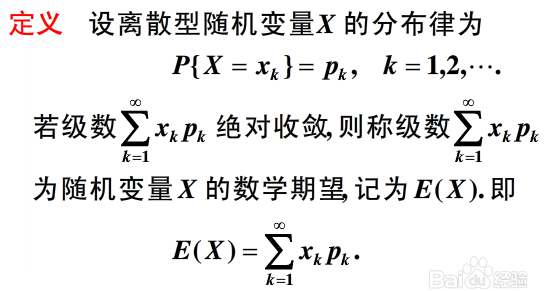（2）连续型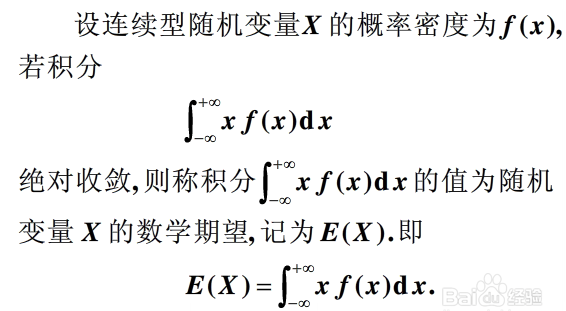3.例题

假设我们来玩一个游戏，一共52张牌，其中有4个A。我们一元钱赌一把，如果你抽中了A，那么我给你10元钱，否则你的一元钱就输给我了，求你赢钱的数学期望。

解：P（抽中A）= 4/52 =1/13

P（抽不中A）= （52-4）/ 52 = 12/13

E（赢钱）= 1/13 * 10 + 12/13 * (-1) = -2/13

即你玩了很多把之后，会发现自己输钱的概率比较高。

参考资料

https://jingyan.baidu.com/article/9f63fb91818ecbc8400f0ee4.html


展开全文• 一、通用公式【数学期望】 1》求解数学期望 2》数学期望的性质 二、常用分布的期望与方差 1》精简版： 2》叨叨版：
一、通用公式【数学期望】

1》求解数学期望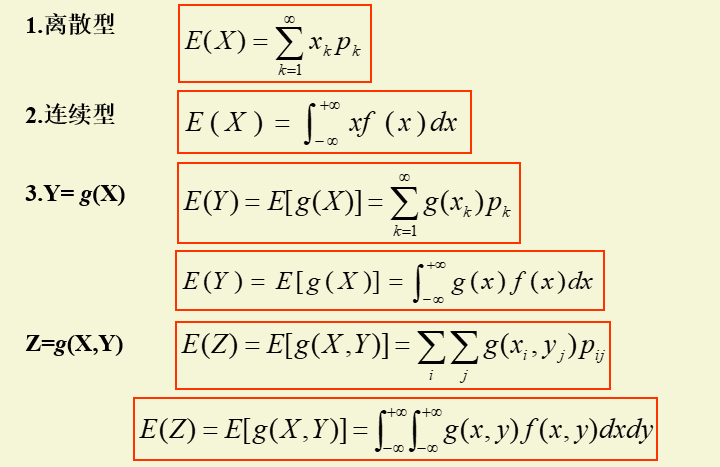2》数学期望的性质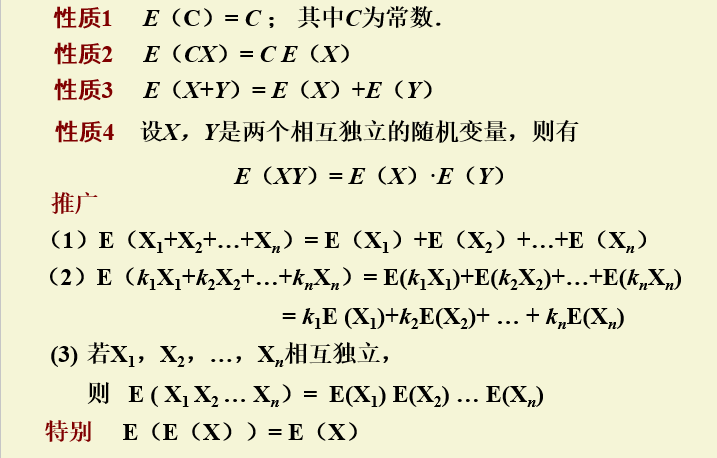二、常用分布的期望与方差

1》精简版：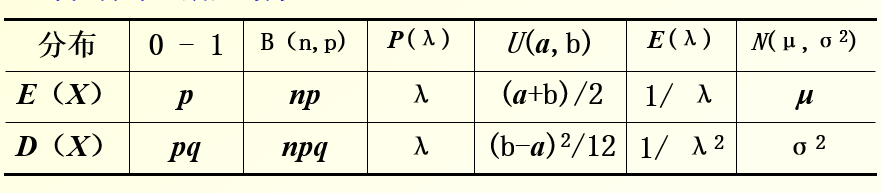2》叨叨版：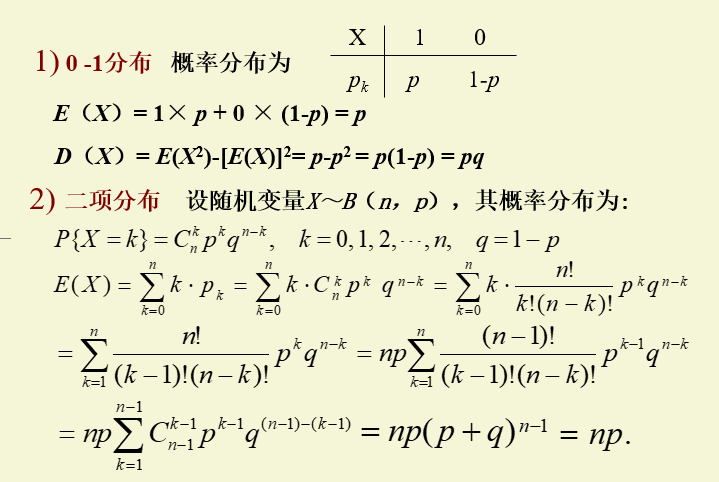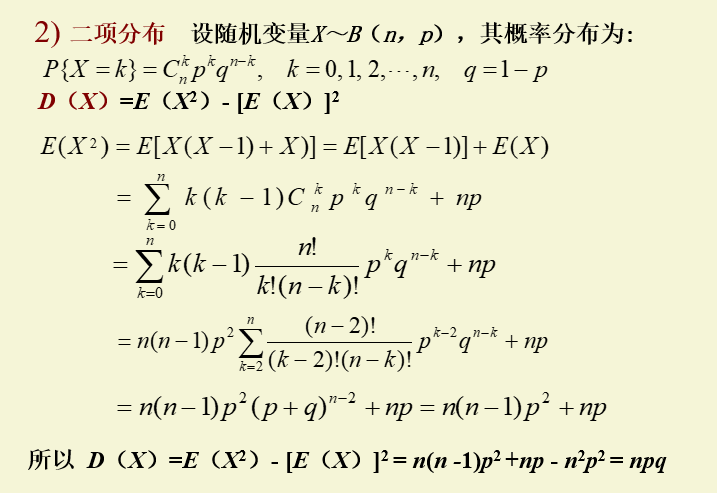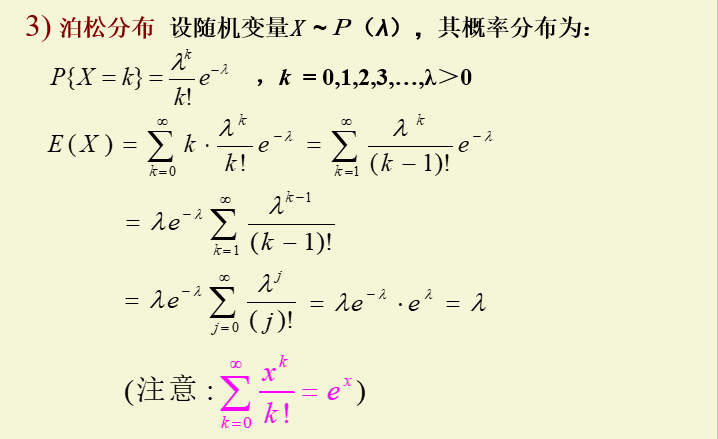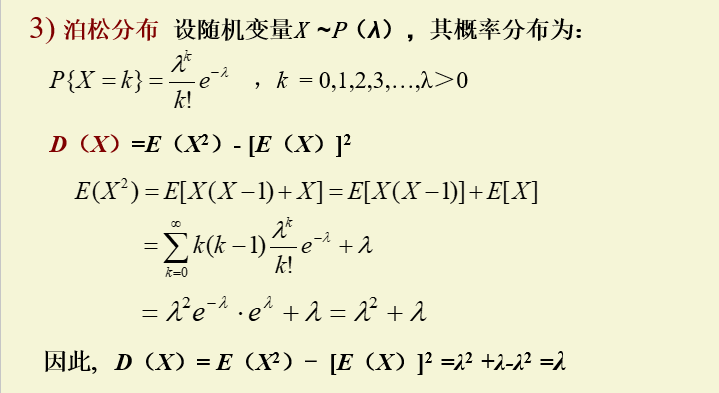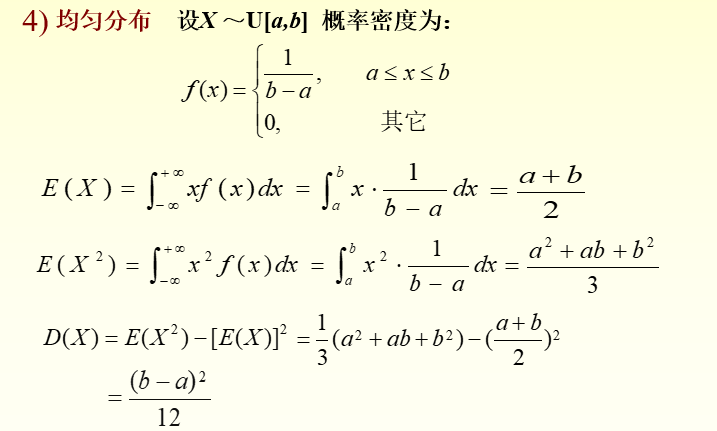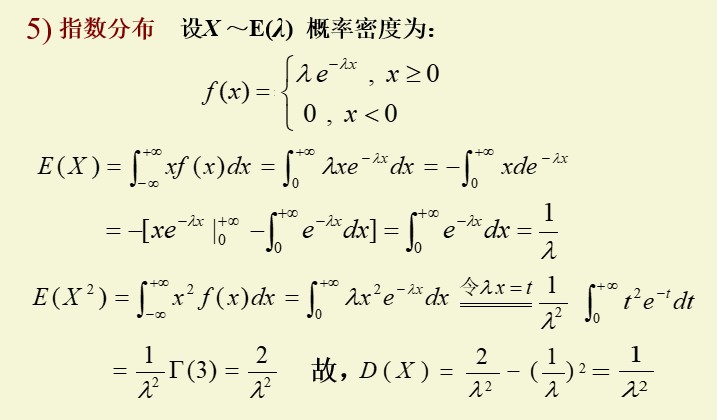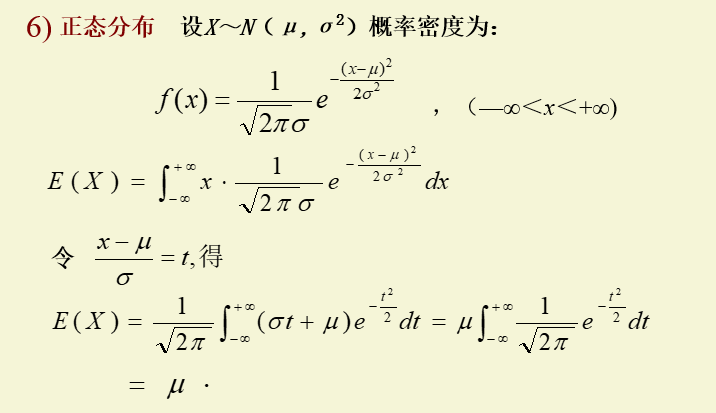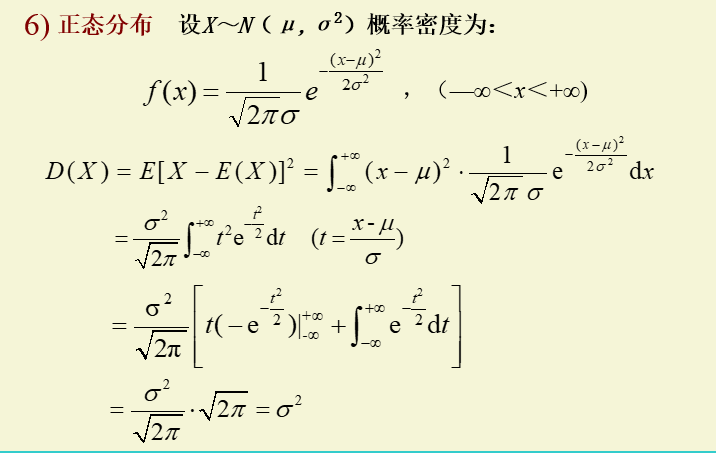展开全文• ## 聊聊数学期望

千次阅读 多人点赞 2019-06-03 23:01:00
因为大家都知道, 每一张彩票的利润的数学期望是负数. 比如真就有这么一种彩票, 他有100k种情况, 只有一种情况可以中奖, 奖金是100k元人民币, 每注两元钱. 为了严谨, 这里加一些限定条件, 假设所有彩票是提前打印好...


故事一

如果把彩票的每一种可能都买一注, 那么不就一定能中奖吗? 确实如此, 但为啥没人这么干呢?

因为大家都知道, 每一张彩票的利润的数学期望是负数.

比如真就有这么一种彩票, 他有100k种情况, 只有一种情况可以中奖, 奖金是100k元人民币, 每注两元钱.

为了严谨, 这里加一些限定条件, 假设所有彩票是提前打印好的100k份, 顾客拿光即完, 不存在2注相同的彩票情况.

我现在把所有的情况每样买一张, 共花了100k*2=200k元人民币, 现在就是用200k元去博它100k元, 平均到每一张彩票上, 就是花两块钱买一张彩票最终只得到一块钱的回报, 我这一张彩票赚了-1元.

所以说, 假如我只买一张彩票, 在结果尚未刮出之前, 根据数学期望, 我就已经亏了1元. 所以我从不买彩票.

启示

在赌博中, 人们有一种"见好就收"的心理, 明知道"久赌必输"的道理, 仍然希望能在前面几次赌博中赚到大钱, 之后再也不赌博了, 认为这样就占到便宜了. 这种由懒惰造成的愚蠢想法在生活中经常出现, 与其在负期望的尝试中碰运气, 不如在正期望的尝试中不断努力, 通过"大数定律"来获得有意义的投资回报. 上帝不掷色子, 虽然生活中处处存在偶然, 但整体上一定是必然的:在随机事件的大量重复出现中，往往呈现几乎必然的规律, 这就是大数定律.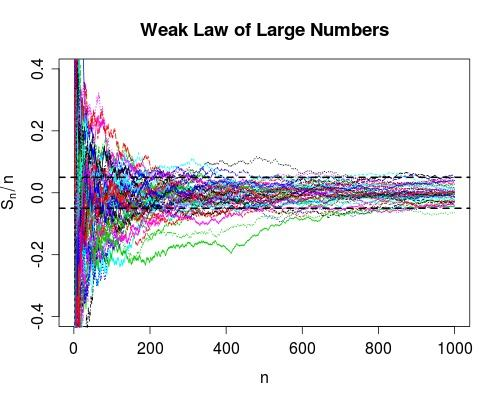故事二

17世纪，法国数学家帕斯卡收到一个问题：甲乙两个机器人猜拳，所以他们两人获胜的机率相等，比赛规则是五局三胜(先胜3局者为赢家)，不考虑平局(即每局必出胜负), 赢家可以获得100法郎的奖励。前三局结束时，甲胜了2局，乙胜了1局，这时由于某些原因中止了比赛，那么如何分配这100法郎才比较公平？

一种很"公平"的办法就是利用计算机的随机种子模拟500次接下来2局的情况, 统计2人胜利的次数之比, 按照这个比率来分配100法郎.

不过用概率论的知识，不难得知，甲获胜的可能性大，乙获胜的可能性小。因为甲输掉后两局的可能性只有(1/2)×(1/2）=1/4，也就是说甲赢得最终胜利的概率为=3/4，甲有75%的期望获得100法郎；而乙只有剩下25%的期望获得100法郎奖金。

可见，虽然不能再进行比赛，但依据上述可能性推断，甲乙双方最终胜利的客观期望分别为75%和25%，因此甲应分得奖金的100*75%=75(法郎)，乙应分得奖金的的100×25%=25(法郎)。这个故事里出现了“期望”这个词，数学期望由此而来。

启示

宇宙中没有真正意义上的随机数, 局部出现的, 机器生成的都是伪随机数.

用一句话来概括的话, 数学期望值是随机变量在时间这个维度上的加权平均数.故事三

在你面前俩按钮, 红色按下立即获得100w, 绿色按下50%几率获得1个亿, 剩下50%几率啥也没有...你会怎么按?

先说答案, 我会按绿色按钮来赌1个亿.

为了严谨一些, 这个故事也加一点条件, 首先, 幸福指数与金钱数量成正比, 也就是硕100w可以获得100w点幸福值, 1亿元可以获得1亿点幸福值(好罪恶啊...), 然后为啥会出现2个按钮呢? 这个抽奖的机会可以当做是是人生中某一个小概率事件吧.

如果按照期望来评判的话, 红色按钮的期望利润就是100w, 而绿色按钮是5000w, 对不对? 不过有的同学会说, 如果你按了绿色没中怎么办, 不是很可惜, 所以应该选择稳妥的100w? 对不起, 上帝不掷骰子, 今天你碰到这个抽奖的机会, 以后还有可能第二次碰到,  当你第三次再次遇到这个选择抽奖机会的时候, 你一定会非常后悔之前两次都选择了红色按钮!!因为3次选择中拿出一次来赌1个亿是非常值得的.

启示

不要在没有意义的事情上浪费太多时间, 就上上面选择按钮的问题, 果断一点直接从上帝视角观察两个选项的数学期望(数学期望揭示了偶然中存在的必然), 陷入选择困难症让你得不偿失.

不愿意承担风险赚不到大钱, 而且日后一定会后悔. 如果一个人眼光总是局限在当下, 相信局部的伪随机现象而不信大数定律的必然趋势, 他必然会活的平庸而计较, 永远被穷人思维困扰.[还是要学好数理化啊..]日记

《三体》第一部中有一段挺有意思的, 说:

"(绍琳)在教学中把大部分物理定律和参数都改了名字,

欧姆定律改叫电阻定律;

麦克斯韦方程改名成电磁方程;

普朗克常数叫成了量子常数"

"所有的科学成果都是广大劳动人民智慧的结晶, 那些资产阶级学术权威不过是窃取了这些智慧."

感觉大刘这段描述引起舒适, 数学和物理领域有太多的定律和现象都以人名命名, 破坏了科学的"自然"性, 而且句TM难记, 如果像文中这样都以定理本身所描述的对象或者相关的事物来命名, 才会使这些理论变得更好理解, 更"通用".


展开全文平均值 大数定律 随机数
• ## 随机变量的数学期望

万次阅读 多人点赞 2018-11-09 20:02:17
离散型随机变量的数学期望 连续型随机变量的数学期望随机变量
• dp求数学期望时往往是在上一个状态求完期望后，加上新状态的要求再求期望,但每次求dp时所乘的所有概率要等于1 https://www.cnblogs.com/hua-dong/p/8166093.html 数学期望 P=Σ每一种状态*对应的概率。 因为不...
• 数学期望真是一个神奇的东西 又连刷了一波题后 我总结了一下几点TIPs 1.数学期望DP 可以抽象理解为 有向图的DP 概率为边权 每次应该加上点权 类似于这样一幅图 2.最好首先确定到底是起点一定还是终点一定 然后...
• ## 数学期望 Expectation

千次阅读 2017-05-28 18:55:33
机器学习中涉及到的很多概念都和 Expectation 相关联，本文对 数学期望展开进行讨论机器学习
• 数学期望的定义 数学期望的计算公式 例题 1.数学期望的定义 在概率论和统计学中，数学期望（或均值）是试验中每次可能结果的概率乘以其结果的总和，是最基本的数学特征之一。它反映随机变量平均取值的大小。 ...
• ## 数学期望计算方法

千次阅读 2018-12-01 09:20:04
经常遇到关于数学期望的题目，一直不太清楚怎么计算。 昨天好好看了下，在此记录一下。 在概率论和统计学中，数学期望(mean)（或均值，亦简称期望）是试验中每次可能结果的概率乘以其结果的总和，是最基本的数学...
• 数学期望，简单的说，随机变量X的数学期望EX就是所有可能值按照概率加权的和。 例如，一个随机变量有1/2的概率等于1，1/3的概率等于2，1/6的概率等于3，则这个随机变量的数学期望为1*1/2 + 2*1/3 + 3*1/6 = 5/3.非...算法
• 在概率论和统计学中，一个离散型随机变量的数学期望是试验中每次可能结果的概率乘以其结果的总和。 在信息学竞赛中，期望值问题大多是求离散型随机变量的数学期望，如果 X 是一个离散的随机变量，输出值是 x1,x2,.....
• 数学1——概率与数学期望 本文作者frankchenfu，blogs网址http://www.cnblogs.com/frankchenfu/，转载请保留此文字。 1、什么是数学期望数学期望亦称期望、期望值等。在概率论和统计学中，一个离散型随机变量...
• ## 数学期望就是均值

千次阅读 2019-03-14 21:10:47
数学期望=np（p为概率，n为集合数） 均值=！平均值math
• 在描述统计当中，数学期望通常是均值 概率论中，其实也没有多大差别，数学期望表达的是事件在概率确定时最终发生的期望值。 这里有一个思维转换，为何均值也是期望值呢？需要联系一下回归的公理论证，譬如切比雪夫...
• 数学期望一、什么是期望二、引入三、一些小性质 一、什么是期望 在概率论和统计学中，数学期望(mean)（或均值，亦简称期望）是试验中每次可能结果的概率乘以其结果的总和，是最基本的数学特征之一。它反映随机变量...
• ## 机器学习-数学期望

千次阅读 2017-11-16 11:55:42
1.什么是数学期望 在概率论和统计学中，数学期望(mean)（或均值，亦简称期望）是试验中每次可能结果的概率乘以其结果的总和 严格的定义如下： 2.数学期望的含义 这个很重要，我们一定要明白概念的...
• ## 浅谈数学期望

千次阅读 2019-07-27 19:14:00
数学期望当前在OI中是一个类似于数论方面门槛的知识，在竞赛中有考察。本文将详细的讲解此内容，但也不是只纠缠于简单的概念，而会解决一些题目.可能这样介绍的知识对于大佬来说还是比较基础，但对像我这样的萌新来...
• 离散型与连续型随机变量的数学期望的定义（二项分布的期望） 推到过程（本人也不是很明白这位大佬在干嘛） （不过他的过程不难理解） （几何分布的期望） 伽马分布的期望 方差 重点经常用的 一个期望公式 ....
• 数学期望 数学期望其实就是加权平均数。 P(X=Xk)=Pk,如果∑k=1nxkP(Xk)收敛，则E(X)=∑k=1nxkP(Xk)为X的数学期望。P(X = X_k) = P_k, 如果\sum_{k=1}^{n}x_kP(X_k)收敛，则E(X) = \sum_{k=1}^{n}x_kP(X_k)为X的数学...
• 题目描述小明同学最近学习了概率论，他了解到数学期望的定义：设X为一个随机变量，X可以取n种不同的取值x1,x2,x3,…,xn。取x1的概率为p1,取x2的概率为p2,以此类推。定义随机变量X的数学期望为：E[X]=x1*p1+x2*p2+…+...算法...